IT

# Announcing Smarter Real-Time Alerts With the KPSS Statistic for Signalflow

Last year we released Built-in Alert Conditions, an open-source library of SignalFlow programs capturing common analytical strategies for detecting anomalies. By experimenting with a set of interpretable parameters (e.g., duration, percentage, number of standard deviations), users are able to tune alerts to their environments without being experts in the underlying statistical methodology. The alert conditions make the power and flexibility of the SignalFlow language more broadly accessible and help power many detectors across a wide range of our customers’ use cases.

The selection of an alert condition is sometimes straightforward: a user should know if a signal represents a resource (e.g., disk) whose running out requires attention, or if a signal failing to report should raise an alarm. On the other hand, how to choose between a Sudden Change and Historical Anomaly alert is less obvious. Does it make sense to compare current values of a signal to the values observed last week (Historical Anomaly), or would the values from the preceding hour provide a better baseline (Sudden Change)?

With an eye towards this question, we are proud to announce the addition of the Kwiatkowski–Phillips–Schmidt–Shin (KPSS) tests for stationarity and trend-stationarity to SignalFlow.

## What is Stationarity, and Why Should You Care?

A time series is stationary if the distribution of its values does not change over time. This implies statistics, calculated over sufficiently large windows, should not change significantly. On a chart, a stationary time series will appear as a roughly horizontal line, possibly altered by some jitter.

Time series with a trend or seasonal component, by contrast, will fail to be stationary. In this post we will focus on the test for stationarity, but we note that the KPSS statistic for trend-stationarity can be used to detect signals that are stationary around a simple linear trend.

The stationarity of a signal has implications for alerting schemes. If a signal is stationary, understanding the tails of its distribution should guide the selection of thresholds for alerts. If one is not interested in inspecting histograms to decide thresholds, a Sudden Change alert (comparing the past five minutes, say, to the preceding hour of data) is sensible. If a signal possesses a trend, a more sophisticated time series model such as double exponential smoothing is likely appropriate. If, for example, a signal has a weekly seasonality, a Historical Anomaly alert will produce the best results. This logic is summarized in the following decision tree.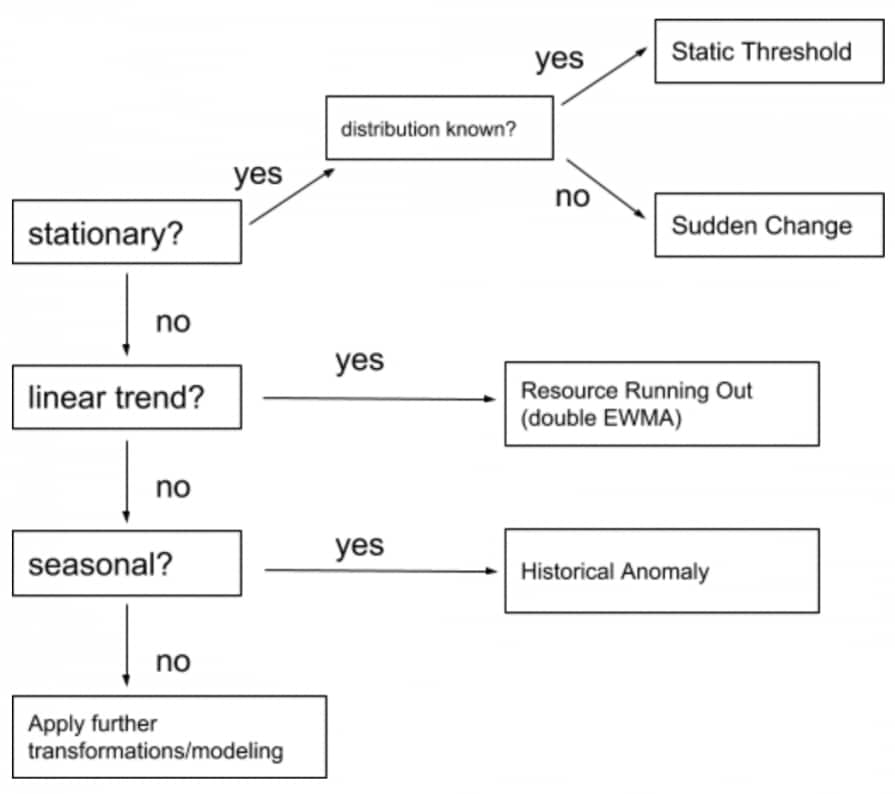This diagram does not cover the scenario in which we use a Static Threshold alert to enforce a business requirement. For signals directly related to an SLA or latency, for example, we may wish to be notified (that we are not serving requests quickly enough) independent of any statistical properties. Also, the goal of “further transformations/modeling” is to place ourselves in one of the classes (stationarity, linear trend, seasonal) we know how to accurately model and alert on.

## A Brief (Technical) Introduction to the KPSS Statistic

The KPSS test has its roots in econometrics. The original paper (referenced below) defined the statistic, studied its distribution, and applied it to some macroeconomic time series (in conjunction with a unit root test).

The KPSS statistic is defined as follows. Suppose x1, …, xn are the datapoints of some slice of a time series. We assume the series can be written xt = rt + 𝜀t, where rt = rt-1 + ut is a random walk and 𝜀t is stationary noise, and ut and 𝜀t are normally distributed with mean zero (in particular, neither has any time dependence). The statistic tests the hypothesis that the variance of the ut’s is zero. If this holds, the series will differ only noisily from the level r = r1 = = rn.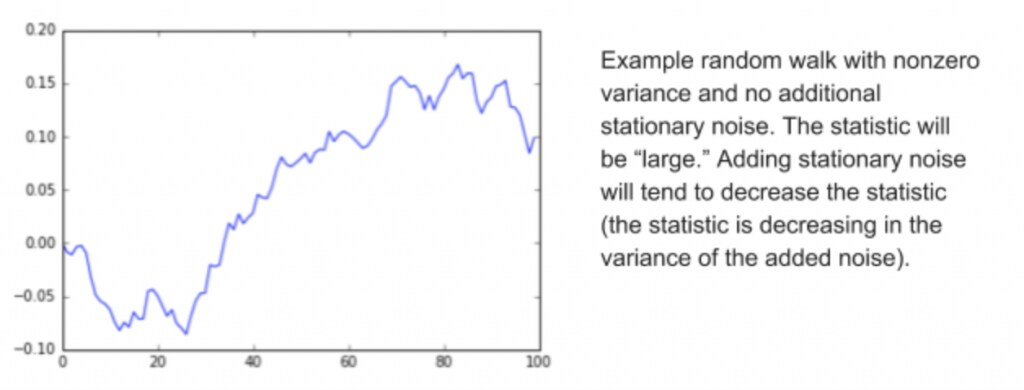This works out as follows. Let 𝜇 denote the mean (x1 + … + xn)/n of the series, and let e1 = x1 – 𝜇, , en = xn – 𝜇 denote the residuals. Now consider the sequence of partial sums of those residuals: s1 = e1, s2 = e1 + e2, …, sn = e1 + e2 + + en. The KPSS statistic is then the sum s12 + s22 + + sn2, appropriately normalized so that it does not depend on the scale of the original data. If the original series oscillates noisily (in particular, in a time-independent way) around its mean, the residuals should never accumulate. If the deviation from the mean has any dependence on time, some of the partial sums will very likely be larger than expected.

## Using KPSS in SignalFlow

SignalFlow now includes a new stream method, aptly called kpss, which has a syntax similar to other time-based transformations. Here is a sample application; additional documentation is available.

kpss_1h = data(‘cpu.utilization’).kpss(over=’1h’) # statistic over the last hour
kpss_smoothed = kpss_1h.percentile(50, over=’1d’) # typical 1-hour statistic over last day

Since the statistic involves a sum of squares, it is influenced by extreme values. Using a percentile of the statistic mitigates that influence. For example, if a signal is mostly stationary but experiences a single (very temporary) spike during a day, that spike will only participate in the rolling 1-hour window for just over two hours, so will not influence the signal kpss_smoothed.

While the assumptions of the KPSS paper (i.e., the assumptions under which the distributions are analyzed) may not apply to every time series that arises in monitoring, and using the KPSS statistic by itself might not be adequate for publishable econometric analysis, the statistic is a step towards characterizing a signal’s behavior. To get a feel for what the statistic is measuring and what sorts of behaviors it can distinguish, consider the following two signals.

## Example of a Stationary Signal

The first is the cache hit ratio, i.e., cache hits divided by the sum of cache hits and cache misses. While cache hits and cache misses themselves have a rough weekly seasonality (since the volume of queries against this particular database depends in part on user activity), the cache hit ratio turns out to be more or less stationary.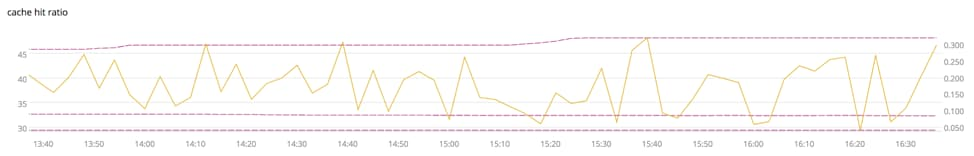The orange line is the cache hit ratio, viewed over the last 3 hours. It is plotted against the left axis. We can see it jumps around a bit, but does not have any obvious trend or seasonality. Calling that cache_hit_ratio, the dashed pink lines correspond to cache_hit_ratio.kpss(over=’1h’).percentile(pct, over=’1d’) for pct=10, 50, 90. These are plotted against the right axis.

Note the KPSS calculation depends on the last 25 hours of data, whereas the chart shows only the last 3 hours of data. We view the signal cache_hit_ratio.kpss(over=’1h’).percentile(50, over=’1d’) as answering the question: over the past day, how stationary is a typical hour? The right axis shows a typical hour (sampled every 3 minutes) has a stationarity score of around 0.08. The 90th percentile answers: over the past day, how stationary are the vast majority of hours.

As discussed earlier, if a typical hour is stationary, two alert types suggest themselves: a Sudden Change alert, for example detecting when the most recent five minutes look very different from the preceding hour; or a Static Threshold alert, where the threshold is set based on prior experience, or an investigation of the chart.

## Example of a Non-Stationary Signal

The second signal, the total number of analytics jobs running across our production cluster, has a rough weekly seasonality. The value of a Static Threshold alert is therefore limited, as the range of normal values depends heavily on the time of day and day of week. For a signal like this, the Historical Anomaly alert is appropriate.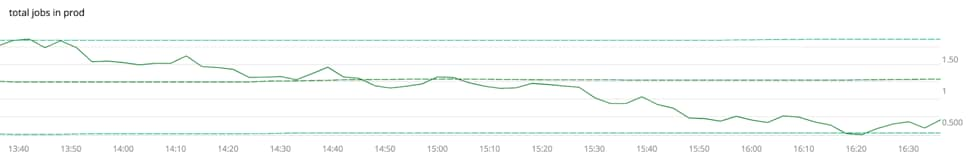The solid line shows the last 3 hours of the total number of jobs signal, during the descent following the midday peak. The dashed lines show the percentiles of the 1-hour KPSS statistics as in the previous example. We see a typical hour (sampled every 3 minutes) has a stationarity score of around 1.15.

The statistic can be thought of as a stationarity “score” (the closer to 0, the more stationary), and it nicely distinguishes the signal that appears to hover around a fixed value from the one whose values vary with the time of day. How should we interpret these values?

## Guidelines for the KPSS statistic

We propose some rough guidelines for the statistic, with the goal of identifying those signals for which a Static Threshold or Sudden Change alert is appropriate (the initial split in the decision tree diagram above).

Both example calculations are performed on 1-hour windows, sampled every 3 minutes, meaning the statistic is calculated on a time series of length 20. We do not recommend calculating on series much shorter than this; the experiments from the original KPSS paper vary the length of the series from 30 to 500. As in the examples, considering the 1-hour statistics over a longer window will reduce the influence of outliers. Accordingly, we will assume the KPSS statistic is calculated on series with lengths in the range of 20 to 50.

For the purposes of alert creation, we suggest broad guidelines as follows: time series with statistic less than 0.6 may be considered stationary; those in the range 0.6 to 1.0 are somewhat ambiguous; those above 1.0 may be considered non-stationary. See the final section for a justification of these ranges.

## Using Stationarity to Select an Alert Condition

For the cache hit ratio example, it makes sense to alert when the ratio is “too low” relative to a baseline; this may affect performance and could be explained by, for example, a change in query patterns or a code push. The stationarity statistic suggests a Sudden Change alert (i.e., using recent history to calculate a baseline) rather than a Historical Anomaly alert (using data from windows spaced, say, one week apart).

Using the Alert Preview feature in SignalFx, we find the Sudden Change condition produces zero alerts over the past several days, whereas the Historical Anomaly condition produces an alert.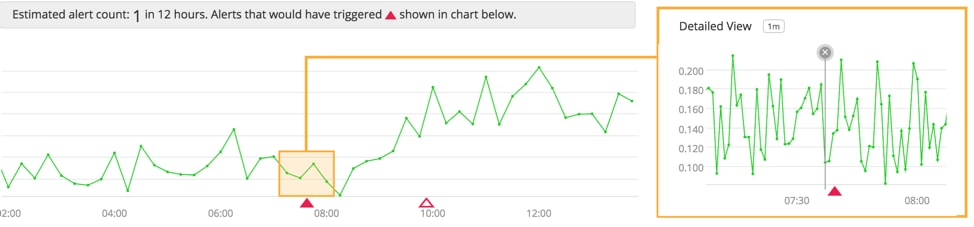Inspecting the timeshifted signal around the time of the (simulated) alert, we observe the signal is generally stationary, and it happens to have been stationary at a different (higher) level in preceding weeks.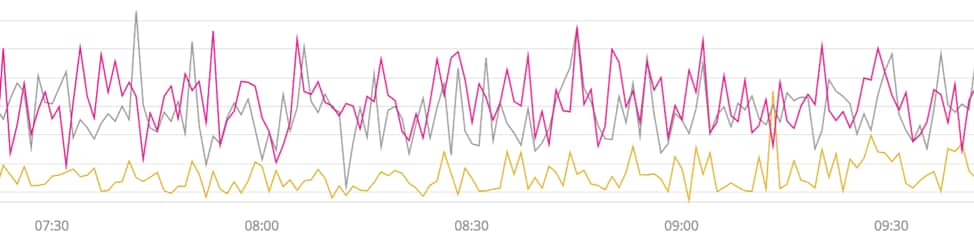The Historical Anomaly alert is picking up on this discrepancy, but the general character of the signal is such that recent history is typically much more predictive of the present than is data from exactly 1, 2, 3, etc. week(s) ago. Viewing 2 weeks of data, we can see the signal changes among states, but does not have a weekly seasonal pattern. (The drops that appear sudden turn out to be much more gradual when the data is viewed at a finer resolution.)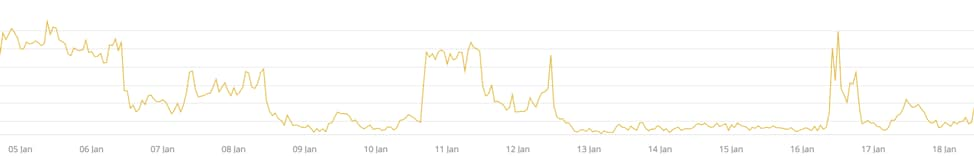By allowing the KPSS statistic to guide our selection of the alert condition, we eliminate a low-quality alert.

## Towards Streaming Classification: An Incremental-Decremental Rolling Window Implementation

Transformations in SignalFx calculate various statistics on a rolling window. The computation alternates between removing the influence of the oldest point in the window (e.g., if we are calculating a rolling sum, subtracting the oldest point from the sum) and incorporating the newest point. Maintaining a rolling window is one way to ensure that stale data is discarded and thresholds for alerts are kept fresh; this is particularly important for monitoring rapidly changing computing environments.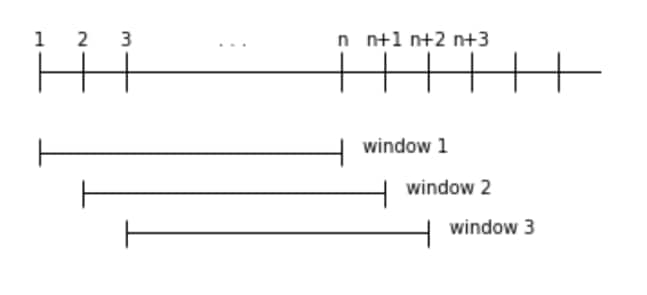For performance and resource reasons, both the computation required for each removal (“decrementing”) and addition (“incrementing”), and the memory overhead of the whole computation, must be independent of the window size. In this setting, we are trying to calculate the KPSS statistic on the windows {x1, …, xn}, {x2, …, xn+1}, {x3, …, xn+2}, … Since the mean of the window changes as it rolls, the residuals and their partial sums change as well.

Fortunately, we are able to avoid recomputing over the entirety of the window as it rolls. By some algebraic machinations, we isolate the contribution of the oldest point and efficiently remove its influence from the computation. Similarly, we can efficiently perform an update when a new point arrives. The procedure is more involved than maintaining a rolling mean or variance as the KPSS statistic is sensitive to the ordering of the points in the window, whereas many rolling statistics are not.

While the KPSS statistic is typically used to conduct detailed (offline) econometric modeling, our incremental-decremental implementation allows for it to be incorporated into our streaming analytics engine. Since the statistic provides a measure of the stationarity of a signal, this is a step towards streaming time series classification.

## Trend-Stationarity KPSS Statistic in SignalFlow

For completeness, we briefly discuss the trend-stationarity variant of the KPSS statistic, which is also supported in SignalFlow. The model is xt = 𝛼t + rt + 𝜀t, and in the statistic the residuals et = xt – 𝜇 are replaced by the residuals of regressing the xt’s against a trend and intercept. (Of course the mean can be viewed as the solution to regressing the series against an intercept only.) This too can be calculated in an incremental-decremental fashion, and along the way, we obtain an incremental-decremental implementation of linear regression of a time series against trend and intercept terms.

To access this variant, supply the kpss method with the keyword argument mode with value ‘trend’. To obtain the 1-hour trend-stationarity statistics of the stream s, for example, we would publish s.kpss(over=’1h’, mode=’trend’). The possible values for the mode argument are ‘level’ and ‘trend’, with ‘level’ being the default. Note that the guidelines for interpreting the trend-stationarity statistic are different from those for interpreting the stationarity statistic, and we will not discuss them here.

## Further Discussion on Interpreting Values of the KPSS Statistic

The first signal (cache hit ratio) appears roughly stationary. A typical hour has a stationarity score of around 0.08, and the band constructed in the chart says the interdecile range is roughly 0.04 to 0.3.

The second signal (analytics jobs across cluster) has a weekly seasonality and is not stationary, even on 1-hour windows. A typical hour has a stationarity score of around 1.15, and the interdecile range is roughly 0.32 to 1.78. For the 3 hours of data shown, the statistic has an interdecile range of roughly 0.9 to 1.75.

For context, for time series with 20 points, the KPSS statistic has the following general behavior.

• Independent samples from pretty much any fixed distribution: 0.13
• Level change (10 readings at value1 followed by 10
readings at value2, plus noise that is small relative to the difference value2 – value1,
if desired): 1.68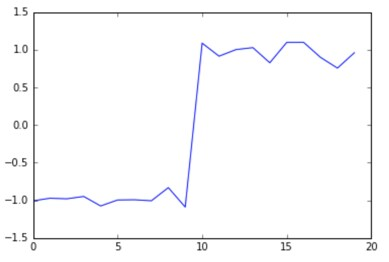• Simple linear trend, arbitrary slope and intercept (plus noise if desired): 2.0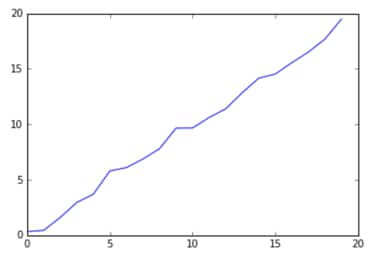For both the level change and simple linear trend, the statistic grows linearly with the length of the time series.

Table 1 of the original KPSS paper provides upper tail percentiles for the stationarity statistic, valid as the window size gets very large. The value 0.574 of the statistic corresponds to the p-value 2.5%, and 0.739 corresponds to 1%. Precisely this means that (under some assumptions), assuming the null hypothesis of stationarity holds, the probability of observing a statistic greater than or equal to 0.739 is 1%. Thus large values of the statistic can be viewed as evidence of non-stationarity, but cannot be directly interpreted as a probability that the series is stationary. Without wading too deeply into the issues surrounding p-values, we hope that the critical values and the calculations on example data together provide a sense of harmony between the visual appearance of stationarity of the cache hit ratio, and a KPSS statistic of 0.08; and between the non-stationarity of the jobs signal, and a statistic of 1.15.

We are excited to see what use cases can be tackled with the aid of the kpss method! Visit our documentation and start using it in your own detectors. If you encounter questions or make some interesting discoveries along the way, feel free to reach out here or @robusteza.

Reference
Kwiatkowski, D., Phillips, P. C., Schmidt, P., and Shin, Y. Testing the null hypothesis of stationarity against the alternative of a unit root. Journal of Econometrics 54, 1-3 (1992), 159–178.Posted by

#### Joe Ross

Joe is a data scientist at SignalFx. Previously Joe worked at other startups and in academia as a professor and researcher in mathematics.
TAGS

Show All Tags
Show Less Tags